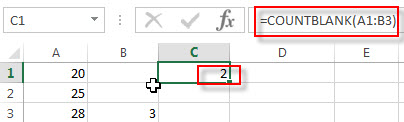# Excel COUNTBLANK Function

This post will guide you how to use the COUNTBLANK function with syntax and examples in Microsoft excel.

### Description

The Excel COUNTBLANK function use to count the number of empty cells in a range of cells. So you can use the COUNTBLANK function to get the number of blank cells in a given range in Excel.

This function will not count the cells that contain text, numbers, error.

The COUNTBLANK function is a build-in function in Microsoft Excel and it is categorized as a Statistical Function.

The COUNTBLANK function is available in Excel 2016, Excel 2013, Excel 2010, Excel 2007, Excel 2003, Excel XP, Excel 2000, Excel 2011 for Mac.

### Syntax

The syntax of the COUNTBLANK function is as below:

`= COUNTBLANK (range)`

Where the COUNTBLANK function argument is:

• Range – This is a required argument.  The range from which you wish to count the empty cells.

Note:

• If one formula that return empty text, and it can be considered blank cell and it will be counted by the COUNTBLANK function.
• If cells contain zero and it will not be considered blank cell, so it will not be counted.

### Excel COUNTBLANK Function Examples

The below examples will show you how to use Excel COUNTBLANK Function to return the number of blank cells in a supplied range of cells.

1# to count empty cells in a given range A1:B3, using the following formula:

`=COUNTBLANK(A1:B3)`### Related Functions

• Excel COUNT function
The Excel COUNT function counts the number of cells that contain numbers, and counts numbers within the list of arguments. It returns a numeric value that indicate the number of cells that contain numbers in a range…
• Excel COUNTA function
The Excel COUNTA function counts the number of cells that are not empty in a range. The syntax of the COUNTA function is as below:= COUNTA(value1, [value2],…)…
• Excel COUNTIF function
The Excel COUNTIF function will count the number of cells in a range that meet a given criteria. This function can be used to count the different kinds of cells with number, date, text values, blank, non-blanks, or containing specific characters.etc.= COUNTIF (range, criteria)…